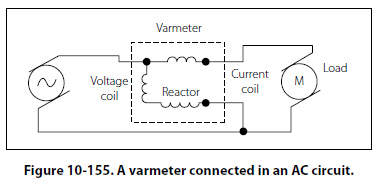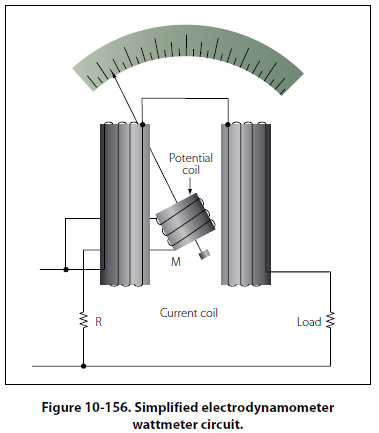Inclined Coil Iron Vane Meter The principle of the moving iron vane mechanism is applied to the inclined coil type of meter, which can be used to measure both AC and DC. The inclined coil, iron vane meter has a coil mounted at an angle to the shaft. Attached obliquely to the shaft, and located inside the coil, are two soft iron vanes. When no current flows through the coil, a control spring holds the pointer at zero, and the iron vanes lie in planes parallel to the plane of the coil. When current flows through the coil, the vanes tend to line up with magnetic lines passing through the center of the coil at right angles to the plane of the coil. Thus, the vanes rotate against the spring action to move the pointer over the scale. The iron vanes tend to line up with the magnetic lines regardless of the direction of current flow through the coil. Therefore, the inclined coil, iron vane meter can be used to measure either alternating current or direct current. The aluminum disk and the drag magnets provide electromagnetic damping. Like the moving iron vane meter, the inclined coil type requires a relatively large amount of current for fullscale deflection and is seldom used in high resistance low power circuits. As in the moving iron vane instruments, the inclined coil instrument is wound with few turns of relatively large wire when used as an ammeter and with many turns of small wire when used as a voltmeter. Varmeters Multiplying the volts by the amperes in an AC circuit gives the apparent power: the combination of the true power (which does the work) and the reactive power (which does no work and is returned to the line). Reactive power is measured in units of vars (volt-amperes reactive) or kilovars (kilovolt-amperes reactive, abbreviated kVAR). When properly connected, wattmeters measure the reactive power. As such, they are called varmeters. Figure 10-155 shows a varmeter connected in an AC circuit.Wattmeter Electric power is measured by means of a wattmeter. Because electric power is the product of current and voltage, a wattmeter must have two elements, one for current and the other for voltage. For this reason, wattmeters are usually of the electrodynamometer type. [Figure 10-156]The movable coil with a series resistance forms the voltage element, and the stationary coils constitute the current element. The strength of the field around the potential coil depends on the amount of current that flows through it. The current, in turn, depends on the load voltage applied across the coil and the high resistance in series with it. The strength of the field around the current coils depends on the amount of current flowing through the load. Thus, the meter deflection is proportional to the product of the voltage across the potential coil and the current through the current coils. The effect is almost the same (if the scale is properly calibrated) as if the voltage applied across the load and the current through the load were multiplied together. If the current in the line is reversed, the direction of current in both coils and the potential coil is reversed, the net result is that the pointer continues to read up scale. Therefore, this type of wattmeter can be used to measure either AC or DC power.
 ©AvStop Online Magazine                                                                                                                                                      Contact Us              Return To Books